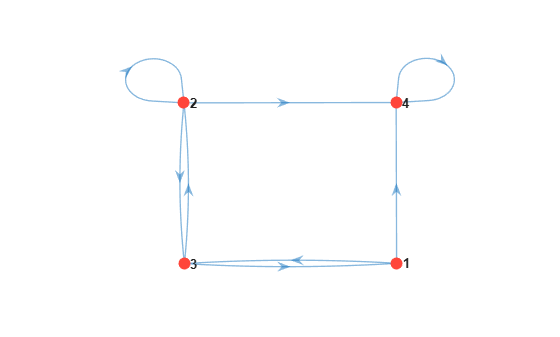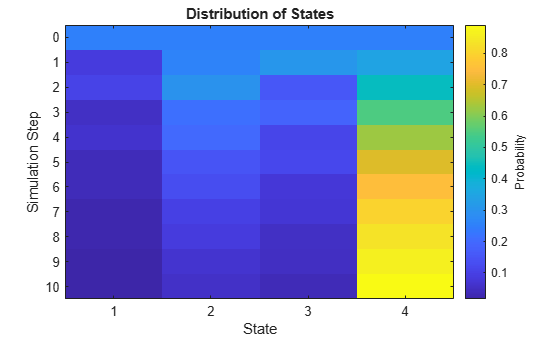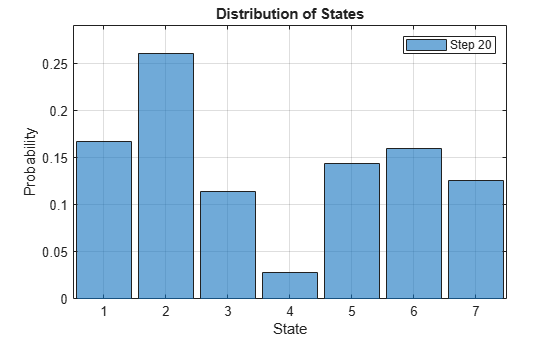# distplot

Plot Markov chain redistributions

## Syntax

``distplot(mc,X)``
``distplot(mc,X,Name,Value)``
``distplot(ax,___)``
``h = distplot(___)``

## Description

example

``distplot(mc,X)` creates a heatmap from the data `X` showing the evolution of a distribution of states in the discrete-time Markov chain `mc`.`

example

````distplot(mc,X,Name,Value)` uses additional options specified by one or more name-value arguments. For example, specify the type of plot or the frame rate for animated plots.```
``` `distplot(ax,___)` plots on the axes specified by `ax` instead of the current axes (`gca`) using any of the input argument combinations in the previous syntaxes.```
``` `h = distplot(___)` returns a handle to the distribution plot. Use `h` to modify properties of the plot after you create it.```

## Examples

collapse all

Create a four-state Markov chain from a randomly generated transition matrix containing eight infeasible transitions.

```rng('default'); % For reproducibility mc = mcmix(4,'Zeros',8);```

`mc` is a `dtmc` object.

Plot a digraph of the Markov chain.

```figure; graphplot(mc);```State `4` is an absorbing state.

Compute the state redistributions at each step for 10 discrete time steps. Assume an initial uniform distribution over the states.

`X = redistribute(mc,10)`
```X = 11×4 0.2500 0.2500 0.2500 0.2500 0.0869 0.2577 0.3088 0.3467 0.1073 0.2990 0.1536 0.4402 0.0533 0.2133 0.1844 0.5489 0.0641 0.2010 0.1092 0.6257 0.0379 0.1473 0.1162 0.6985 0.0404 0.1316 0.0765 0.7515 0.0266 0.0997 0.0746 0.7991 0.0259 0.0864 0.0526 0.8351 0.0183 0.0670 0.0484 0.8663 ⋮ ```

`X` is an 11-by-4 matrix. Rows correspond to time steps, and columns correspond to states.

Visualize the state redistribution.

```figure; distplot(mc,X)```After 10 transitions, the distribution appears to settle with a majority of the probability mass in state `4`.

Consider this theoretical, right-stochastic transition matrix of a stochastic process.

`$P=\left[\begin{array}{ccccccc}0& 0& 1/2& 1/4& 1/4& 0& 0\\ 0& 0& 1/3& 0& 2/3& 0& 0\\ 0& 0& 0& 0& 0& 1/3& 2/3\\ 0& 0& 0& 0& 0& 1/2& 1/2\\ 0& 0& 0& 0& 0& 3/4& 1/4\\ 1/2& 1/2& 0& 0& 0& 0& 0\\ 1/4& 3/4& 0& 0& 0& 0& 0\end{array}\right].$`

Create the Markov chain that is characterized by the transition matrix P.

```P = [ 0 0 1/2 1/4 1/4 0 0 ; 0 0 1/3 0 2/3 0 0 ; 0 0 0 0 0 1/3 2/3; 0 0 0 0 0 1/2 1/2; 0 0 0 0 0 3/4 1/4; 1/2 1/2 0 0 0 0 0 ; 1/4 3/4 0 0 0 0 0 ]; mc = dtmc(P);```

Compute the state redistributions at each step for 20 discrete time steps.

`X = redistribute(mc,20);`

Animate the redistributions in a histogram. Specify a half-second frame rate.

```figure; distplot(mc,X,'Type','histogram','FrameRate',0.5);```## Input Arguments

collapse all

Discrete-time Markov chain with `NumStates` states and transition matrix `P`, specified as a `dtmc` object. `P` must be fully specified (no `NaN` entries).

Evolution of state probabilities, specified as a `(1 + numSteps)`-by-`NumStates` nonnegative numeric matrix returned by `redistribute`. The first row is the initial state distribution. Subsequent rows are the redistributions at each step. `distplot` normalizes the rows by their respective sums before plotting.

Data Types: `double`

Axes on which to plot, specified as an `Axes` object.

By default, `distplot` plots to the current axes (`gca`).

### Name-Value Arguments

Specify optional pairs of arguments as `Name1=Value1,...,NameN=ValueN`, where `Name` is the argument name and `Value` is the corresponding value. Name-value arguments must appear after other arguments, but the order of the pairs does not matter.

Before R2021a, use commas to separate each name and value, and enclose `Name` in quotes.

Example: `'Type','graph','FrameRate',3` creates an animated plot of the redistributions using a frame rate of 3 seconds.

Plot type, specified as the comma-separated pair consisting of `'Type'` and a value in this table.

ValueDescription
`'evolution'`

Evolution of the initial distribution. The plot is a `(1 + NumSteps)`-by-`NumStates` heatmap. Row i displays the redistribution at step i.

`'histogram'`

Animated histogram of the redistributions. The vertical axis displays probability mass, and the horizontal axis displays states. The `'FrameRate'` name-value pair argument controls the animation progress.

`'graph'`

Animated graph of the redistributions. `distplot` colors the nodes by their probability mass at each step. The `'FrameRate'` name-value pair argument controls the animation progress.

Example: `'Type','graph'`

Data Types: `string` | `char`

Length of discrete time steps, in seconds, for animated plots, specified as the comma-separated pair consisting of `'FrameRate'` and a positive scalar.

The default is a pause at each time step. The animation proceeds when you press the space bar.

Example: `'FrameRate',3`

Data Types: `double`

## Output Arguments

collapse all

Handle to the distribution plot, returned as a graphics object. `h` contains a unique plot identifier, which you can use to query or modify properties of the plot.

## Version History

Introduced in R2017b